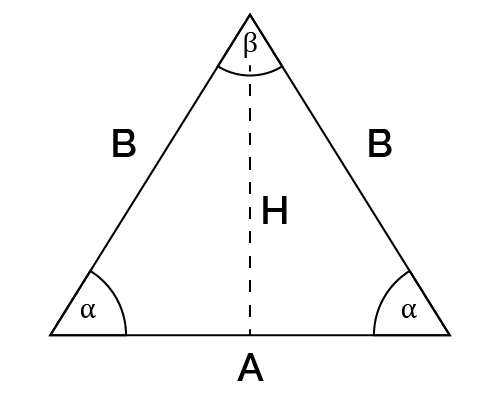# Isosceles Triangle Find A Calculator

Reviewed by Rijk de Wet
Last updated: Jun 20, 2022

Our isosceles triangle find A calculator is exactly what you need to help you to find the base of an isosceles triangle. So if you need a calculator for math or are simply looking to learn more about how we find the base of an isosceles triangle, look no further.

• What is an isosceles triangle;
• What is the base of an isosceles triangle;
• How to find the base of an isosceles triangle; and
• How to use our isosceles triangle, find A calculator.

🙋 An isosceles triangle is a triangle with two equal sides and two equal angles.

## What is the base of a triangle?

When we talk about the base of a triangle, we refer to the side perpendicular to the height. In an isosceles triangle, the base is the side opposite the vertex angle.

## How to use our Isosceles triangle find A calculator

Have you been stumped, asking yourself how to find the base of an isosceles triangle? Our triangle makes your job easy. When you enter the values of the known sides and or angles, our isosceles triangle find A calculator will help you find the base.

Suppose you are aware of the area of the triangle plus the height; you may choose these from the options at the top, then enter these values, and the value of the base will be generated. Alternatively, if you know the vertex angles and the sides labeled $B$, you may choose the vertex angle $\beta$ and side $B$ option to enter these, and our calculator will determine the base's length.

You may also enter the angles of the vertex angle $\beta$, and the sides labeled $B$, or the side $B$ and the height $H$, and our calculator will work out the base.

Note well: You need to reload this calculator before each use.

## How to find the base of an isosceles triangle given the height and side B

To find the base of an isosceles triangle from the height and $B$, we can use a version of the Pythagorean theorem:

$B^2 = \left(\frac{A}{2}\right)^2 + H^2$

where $B$ represents the hypotenuse, $A$ the base, and $H$ the height. As always, we use the Pythagorean theorem with right-angled triangles only. So, to do this, you will need:

1. The triangle's height and the sides that are labeled $B$.
2. In this instance, because $A$ is twice the base of the right-angle triangle, our initial formula will be
$B^2 = \left(A/2\right)^2 + H^2$.
3. Now, let's make $A$ the subject of the formula:
\quad \begin{aligned} \left(\frac{A}{2}\right)^2 &= B^2 - H^2 \\ \frac{A}{2} &= \sqrt{B^2 - H^2} \\ A &= 2 \times \sqrt{B^2 - H^2} \\ \end{aligned}

## How to find the base of an isosceles triangle using the area and the height

To find the base of an isosceles triangle when given the area and height, we use the formula:

$\text{area} = \frac {1}{2} × \text{base} × \text{height}$

When we make the base the subject of the formula, we get:

$\text{base} = \frac{\text{area}} {\text{height}} × 2$

Next, you will need to substitute in whatever values you are given for area and height and solve the equation.

## How to find the base of an isosceles triangle from the apex angle and side B

To find the base of an isosceles triangle, when given the angle of the apex and side $B$, you use the law of cosine or the cosine rule:

\begin{aligned} A^2 &= B^2 + B^2 - 2 (B) (B) \cos\beta \\ A^2 &= 2B^2 - 2 B^2 \cos \beta \\ A &= \sqrt {2B^2 - 2 B^2 \cos\beta} \\ A &= \sqrt {2B^2(1 - \cos\beta)} \\ \end{aligned}

Here is a list of related calculators that may interest you:

## FAQ

### What is the base of an isosceles triangle, given the congruent sides 6 cm and angle of the vertex 80°?

To find the base of an isosceles triangle with a congruent side of 6 cm and base angles of 80 degrees:

1. We will use the formula:
A² = B² + B² - 2(B)(B)cos(β)
2. Substitute in the known values:
A² = 6² + 6² - 2(6)(6)cos(80)
3. Solve the equation:
A² = 36 + 36 - 72 × cos(80)
A² = 72 - 72 × 0.17
A² = 72 - 12.24
A² = 59.6
A = √59.6I know...
Select...
Find A
Base (A)
in
People also viewed…

Addiction calculator tells you how much shorter your life would be if you were addicted to alcohol, cigarettes, cocaine, methamphetamine, methadone, or heroin.

### Complementary angles

The complementary angles calculator determines the complementary angle to the one you input. You can also use this tool to check if two angles are complementary or not.

### Eggs

Quantum physicist's take on boiling a perfect egg.

### LCM

The LCM calculatorcalculates the least common multiple of two to fifteen numbers.